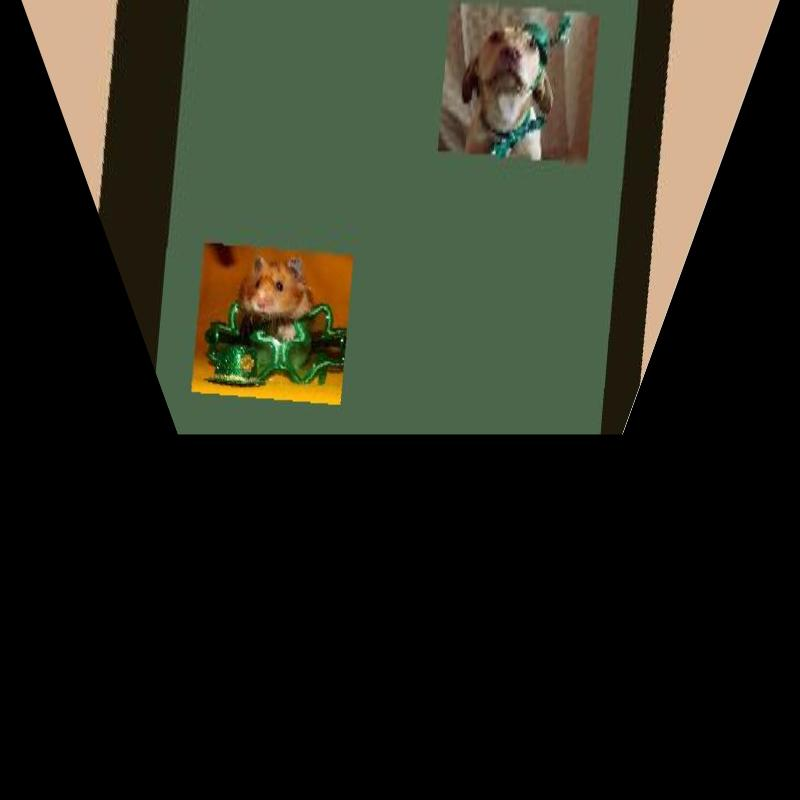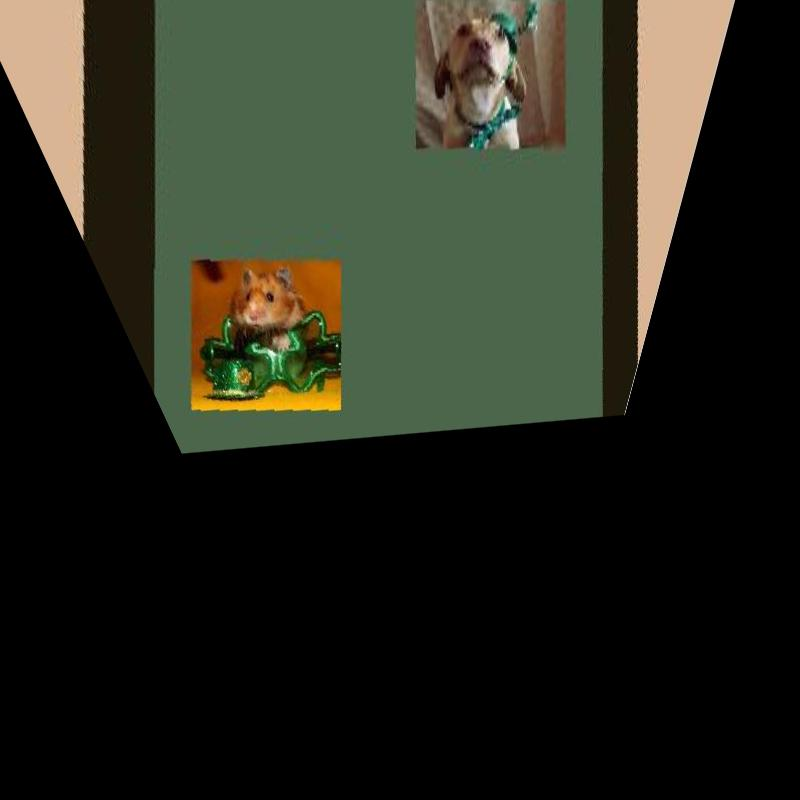# Directed HomographyModel created by script5c.m

```campos([2 23 4]);
camtarget([4 0 1]);
camup([0 0 1]);
camproj('perspective');
camva(40);
```

The camera is looking to the left and down slightly.

Create a video with 90-degree rotation from a model where the camera is parallel to the floor. (see model)

At the end of the sequence we are looking down towards the floor.

Now change the filename to the model above.

At the end of the sequence the floor lines are now diverging rather than converging. We have gone too far.

See published MATLAB in dh1.m

Using information from the model, we can choose an angle wh the floor lines are parallel.See MATLAB published code in dh2.m

The image is still skewed. We can correct this by rotating about the z-axis of the camera. The video sequence below shows the effect of this rotation.

The MATLAB code for this is in dh1a.m Note: we could also have used `imrotate`.

Now we combine the floor-view homography with a rotation, chosen by trial, to produce the image below.The MATLAB code may be found in dh2a.m

Maintained by John Loomis, last updated 28 March 2015